# Fractional evolution equations with nonlocal conditions in partially ordered Banach space

### Abstractcarpathian_2018_34_3_379_390_abstract

### Full PDFcarpathian_2018_34_3_379_390

In the present work, we discuss the existence of mild solutions for the initial value problem of fractional evolution equation of the form

(A)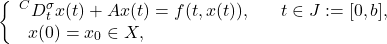where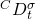denotes the Caputo fractional derivative of order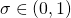,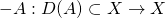generates a positive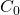-semigroup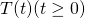of uniformly bounded linear operator in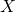,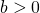is a constant,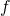is a given functions. For this, we use the concept of measure of noncompactness in partially ordered Banach spaces whose positive cone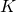is normal, and establish some basic fixed point results under the said concepts. In addition, we relaxed the conditions of boundedness, closedness and convexity of the set at the expense that the operator is monotone and bounded. We also supply some new coupled fixed point results via MNC. To justify the result, we prove an illustrative example that rational of the abstract results for fractional parabolic equations.